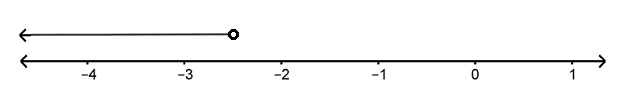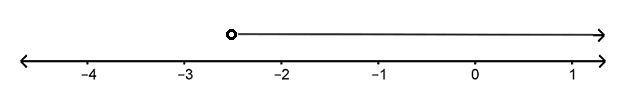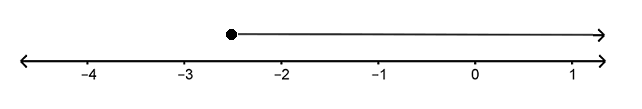# Equations and Inequalities

## Objective

Solve and graph one-step inequalities.

## Common Core Standards

### Core Standards

?

• 7.EE.B.4.B — Solve word problems leading to inequalities of the form px + q > r or px + q < r, where p, q, and r are specific rational numbers. Graph the solution set of the inequality and interpret it in the context of the problem. For example: As a salesperson, you are paid $50 per week plus$3 per sale. This week you want your pay to be at least \$100. Write an inequality for the number of sales you need to make, and describe the solutions.

?

• 6.EE.B.5

• 6.EE.B.8

## Criteria for Success

?

1. Understand that inequalities have infinitely many solutions compared to equations.
2. Understand that a solution to an inequality creates a true statement when the value is substituted into the inequality.
3. Understand how a graph represents the solutions to an inequality.
4. Write and solve one-step inequalities.
5. Draw a number line to graphically represent the solutions to an inequality.

## Tips for Teachers

?

This lesson is approaching 7.EE.4b and reaches back to sixth-grade standards to revisit inequality concepts before students look at two-step inequalities in the upcoming lessons. Students may use number lines as an optional tool in this and the next few lessons to support their understanding of number comparisons and inequality statements (MP.5).

#### Remote Learning Guidance

If you need to adapt or shorten this lesson for remote learning, we suggest prioritizing Anchor Problem 1 (benefits from worked example). Find more guidance on adapting our math curriculum for remote learning here.

#### Fishtank Plus

• Problem Set
• Student Handout Editor
• Vocabulary Package

## Anchor Problems

?

### Problem 1

An airline allows passengers to bring a suitcase that weighs less than 30 pounds onto the airplane for free. If a suitcase weighs 30 pounds or more, then the passenger will be charged a fee. Elena is going on a trip and is packing her suitcase. Her suitcase weighs 4.8 pounds when it is empty.

1. Elena packs 25 pounds in her suitcase and believes that she can safely avoid the fee. Is she correct? Explain your reasoning.
2. Write an inequality to represent the number of pounds that Elena can pack in her suitcase and not pay the fee.

### Problem 2

Which graph below shows all values of ${x \geq -2.5}$?
Which graph below shows all values of ${x< -2.5}$?
For the remaining graph, what inequality represents the solutions shown in the graph?

Graph A:Graph B:Graph C:## Problem Set

?The following resources include problems and activities aligned to the objective of the lesson that can be used to create your own problem set.